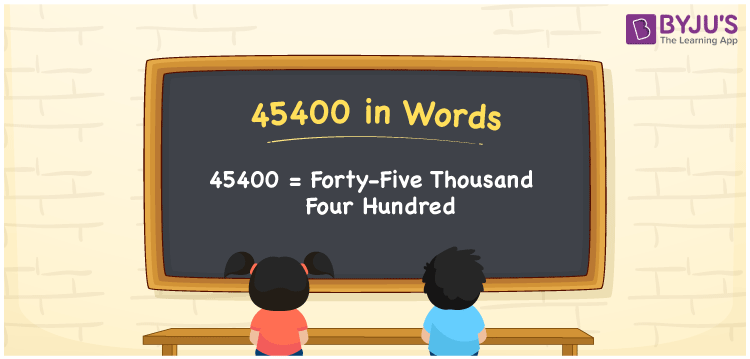# 45400 in Words

In English words, the number 45400 is written as “forty-five thousand four hundred”. The number 45400 is a cardinal number. For example, if you earned Rs. 45400 in 6 months, you can write it as “I earned Rs. Forty-five thousand four hundred in six months”. Now, let us have a look at the procedure of writing the number 45400 using the place value system in this article.

 45400 in Words: Forty-five Thousand Four Hundred. Forty-five Thousand Four Hundred in Numerical Form: 45400.

## 45400 in English Words## How to Write 45400 in Words?

The below table depicts the place values of the five-digit number 45400.

 Ten-thousands Thousands Hundreds Tens Ones 4 5 4 0 0

The expanded form of 45400 is as follows:

= 4 × Ten thousand + 5 × Thousand + 4 × Hundred + 0 × Ten + 0 × One

= 4 × 10000 + 5 × 1000 + 4 × 100 + 0 × 10 + 0 × 1

= 40000 + 5000 + 400

= 45400

= Forty-five thousand four hundred

Hence, 45400 in words is forty-five thousand four hundred.

45400 in words – Forty-five thousand four hundred

Is 45400 an odd number? – No

Is 45400 an even number? – Yes

Is 45400 a perfect square number? – No

Is 45400 a perfect cube number? – No

Is 45400 a prime number? – No

Is 45400 a composite number? – Yes

## Frequently Asked Questions on 45400 in Words

### How to write 45400 in English words?

45400 in words is forty-five thousand four hundred.

### Simplify 45000 + 400, and express it in words.

Simplifying 45000 + 400, we get 45400. Hence, 45400 in words is forty-five thousand four hundred.

### Is 45400 an odd number?

No, 45400 is not an odd number.